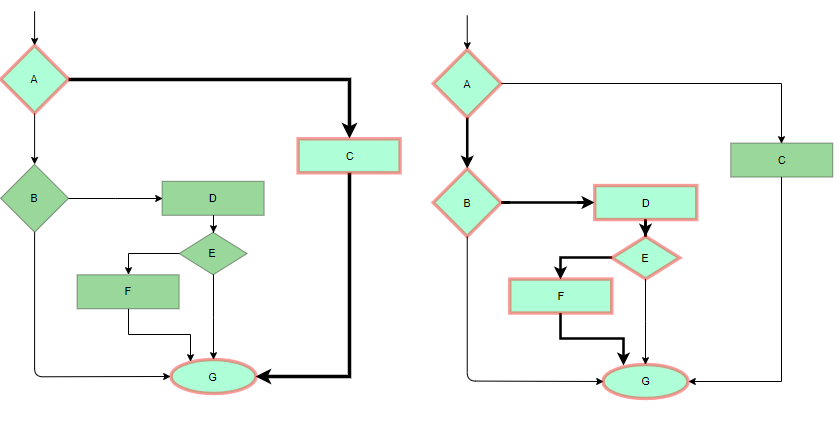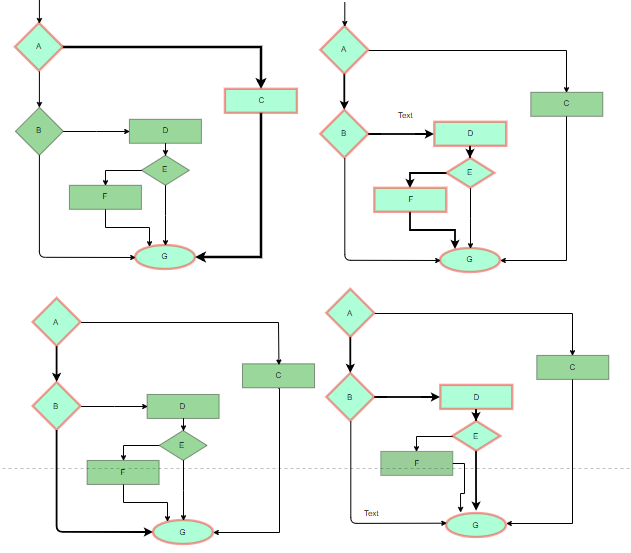Open in App
Not now

# Software Engineering | White box Testing

• Difficulty Level : Easy
• Last Updated : 29 Nov, 2022

White box testing techniques analyze the internal structures the used data structures, internal design, code structure, and the working of the software rather than just the functionality as in black box testing. It is also called glass box testing or clear box testing or structural testing. White Box Testing is also known as transparent testing, open box testing.

Prerequisite – Software Testing | Basics

Working process of white box testing:

• Input: Requirements, Functional specifications, design documents, source code.
• Processing: Performing risk analysis for guiding through the entire process.
• Proper test planning: Designing test cases so as to cover the entire code. Execute rinse-repeat until error-free software is reached. Also, the results are communicated.
• Output: Preparing final report of the entire testing process.

### Testing techniques:

• Statement coverage: In this technique, the aim is to traverse all statement at least once. Hence, each line of code is tested. In case of a flowchart, every node must be traversed at least once. Since all lines of code are covered, helps in pointing out faulty code.Statement Coverage Example

• Branch Coverage: In this technique, test cases are designed so that each branch from all decision points are traversed at least once. In a flowchart, all edges must be traversed at least once.4 test cases required such that all branches of all decisions are covered, i.e, all edges of flowchart are covered

• Condition Coverage: In this technique, all individual conditions must be covered as shown in the following example:
2. IF(X == 0 || Y == 0)
3. PRINT ‘0’
4. #TC1 – X = 0, Y = 55
5. #TC2 – X = 5, Y = 0
• Multiple Condition Coverage: In this technique, all the possible combinations of the possible outcomes of conditions are tested at least once. Let’s consider the following example:
2. IF(X == 0 || Y == 0)
3. PRINT ‘0’
4. #TC1: X = 0, Y = 0
5. #TC2: X = 0, Y = 5
6. #TC3: X = 55, Y = 0
7. #TC4: X = 55, Y = 5
• Basis Path Testing: In this technique, control flow graphs are made from code or flowchart and then Cyclomatic complexity is calculated which defines the number of independent paths so that the minimal number of test cases can be designed for each independent path. Steps:
1. Make the corresponding control flow graph
2. Calculate the cyclomatic complexity
3. Find the independent paths
4. Design test cases corresponding to each independent path
5. V(G) = P + 1, where P is the number of predicate nodes in the flow graph
6. V(G) = E – N + 2, where E is the number of edges and N is the total number of nodes
7. V(G) = Number of non-overlapping regions in the graph
8. #P1: 1 – 2 – 4 – 7 – 8
9. #P2: 1 – 2 – 3 – 5 – 7 – 8
10. #P3: 1 – 2 – 3 – 6 – 7 – 8
11. #P4: 1 – 2 – 4 – 7 – 1 – . . . – 7 – 8
• Loop Testing: Loops are widely used and these are fundamental to many algorithms hence, their testing is very important. Errors often occur at the beginnings and ends of loops.
1. Simple loops: For simple loops of size n, test cases are designed that:
• Skip the loop entirely
• Only one pass through the loop
• 2 passes
• m passes, where m < n
• n-1 ans n+1 passes
2. Nested loops: For nested loops, all the loops are set to their minimum count and we start from the innermost loop. Simple loop tests are conducted for the innermost loop and this is worked outwards till all the loops have been tested.
3. Concatenated loops: Independent loops, one after another. Simple loop tests are applied for each. If they’re not independent, treat them like nesting.

White Testing is Performed in 2 Steps:

1. Tester should understand the code well

2. Tester should write some code for test Cases and execute them

Tools required for White box Testing:

• PyUnit
• Sqlmap
• Nmap
• Parasoft Jtest
• Nunit
• VeraUnit
• CppUnit

1. White box testing is very thorough as the entire code and structures are tested.
2. It results in the optimization of code removing error and helps in removing extra lines of code.
3. It can start at an earlier stage as it doesn’t require any interface as in case of black box testing.
4. Easy to automate.
5. White box testing can be easily started in Software Development Life Cycle.
6. Easy Code Optimization.